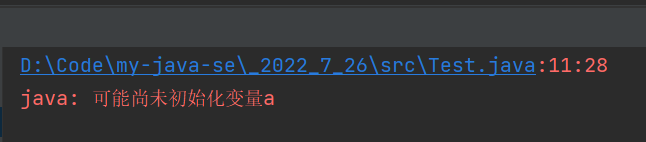﻿ java数据类型与变量的安全性介绍_java_脚本之家
java# java数据类型与变量的安全性介绍

## 一、标识符

1.类名：大驼峰，每个单词首字母大写(HelloWorld)
2.方法名：小驼峰,从第二个单词开始每个单词首字母大写(helloWorld)
3.变量名：小驼峰

• 1.public(关键字)
• 2.520shy(数字开头)
• 3.zhao.d(出现陌生符号)

## 二、数据类型

java当中的整型与浮点数都是严格带有符号位java中有8种基本数据类型1.在java种字符型占2字节，因为在C语言种使用的是ASCII编码,java种使用的是unicode编码方式.
2.不论是在x64,x86环境下，int都占用4字节，long占用8字节.

## 三、变量

### 1.无初始化

``` public static void main(String[] args) {
int a;
System.out.println(a);
}```1.初始化
2.赋值

```public static void main(String[] args) {
int a;
a=10;//1.赋值
System.out.println(a);
int b=10;//2.初始化
System.out.println(b);
}```### 2.赋值超出范围

```public static void main(String[] args) {
int a=2147483648;
System.out.println(a);
}```### 3.long与float的使用• 1.在定义float类型时在数据后面加一个f
• 2.在定义long类型时在数据后面加一个L
```public static void main(String[] args) {
float a=3.5f;
long b=10L;
}```

### 4.除法的使用

```public static void main(String[] args) {
int a=3;
int b=2;
System.out.println(a/b);
}``````  public static void main(String[] args) {
int a=3;
int b=2;
System.out.println(a*1.0/b);
double c=3.0;
double d=2.0;
System.out.println(c/d);
}```### 5.浮点数的精度问题

```public static void main(String[] args) {
double ret=1.1;
System.out.println(ret*ret);
}```### 6.boolean类型变量的使用

```public static void main(String[] args) {
double ret=1.1;
System.out.println(ret*ret);
}```boolean类型的变量只有两种取值，true表示真，false表示假

### 7.类型提升

```public static void main(String[] args) {
int a=2;
long b=3L;
int c=a+b;
}```int与long进行计算时，会把int转换成long，所以存放给int时会报错.

```public static void main(String[] args) {
short a=10;
short b=20;
short c=a+b;
}```CPU通常是按照4个字节从内存中读写数据，为了实现方便，所以低于4字节的类型，会先提升为Int，在计算.

## 四、初始字符串

```public static void main(String[] args) {
String s1="woyao";
String s2="jindachang";
System.out.println(s1+s2);
}```